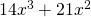# Factoring Expressions with Exponents

Definition: To factor a polynomial is to write the addition of two or more terms as the product of two or more terms.

Consider the addition of the two numbers 24 + 30. Notice that they are both multiples of 6.

We could writeThe factors are ‘6’ and ‘(4+5)’.

Factoring numbers in this way doesn’t mean too much, however changing the form from a sum (the addition of terms) to the product (the multiplication of terms) can be informative for polynomials. Polynomials are introduced on the next page.

What we wish to accomplish on this page is being able to factor an expression of several terms with one, two or possibly three variables.

For example,Greatest Common Factor of Monomials

Taking Common Factor from Binomial

Learning method 2: Open the worksheet below, watch the video, complete the worksheet, check the solutions.

Worksheet: Grade 10 FMP Factor Polynomials

Video: A few examples

Solutions: 10 FMP Factor Polynomial Solutions

Learning method 3: Follow the examples

Example 1: FactorLet’s list the factors of each term:Let’s underline the factors that appear in both terms:Let’s take out of the bracket what is common to both terms, and leave in the bracket what is left from each term:Notice that we took the highest common factor of 14 and 21 out of the brackets, and for the variable, we took the lowest power.

Example 2: FactorNotice that the highest common factor of 15, 20 is 5.

Following the same steps as for example 1 we have:Example 3: Notice the use of ‘1’ in this example. Factor